5.3. Probes of cosmological magnetic field evolution using quasar Faraday rotation statistics

The fact that no Faraday rotation `signal' has yet been detected from a widespread, all-pervading intergalactic plasma (section 5.1), whereas discrete `magnetized cloud systems' have been detected over a similar redshift range (section 5.2), indicates that population models can be constructed for the latter, which can be tested against RM observations of quasars out to significant lookback times in the universe. Welter et a1 (1984) have produced comprehensive models of this type which they have tested against the best RM data available about a decade ago, and concluded that the RM intervenor population shows clear evolutionary effects out to z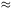2.2. However the data were not sufficient to confidently isolate a particular preferred intervenor model.

The mathematical modeling of discrete sources of RM as a function of z is different from that of the more straightforward case of calculating the `intersection depth' of a sample of intervenors for different cosmological geometries. The latter can be represented as an `optical depth'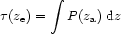(5.6)

where P(za) is the probability of intersection by a cloud which has some number density, n(za) = n0(1 + z)3, and a (dimensionless) cross section, A(za) = cn(za) / H0{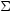0(1 + za)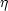}. Here,is the physical cross section of the RM intervenor, whose cosmic evolution we parameterize by, following the notation of Welter et al (1984). The subscript 0 refers to the present epoch. Consequently,(ze) can be written in terms of A0, and making use of equations (5.2) and (5.6), as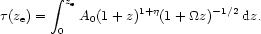(5.7)

This is a purely geometrical term, applicable to the calculation offor any intervenor, whose electromagnetic properties have yet to be specified. If we assume= 0, it integrates easily to become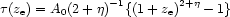(5.8)

Only small numbers of galaxy-scale intersections occur per quasar out to z3.3 (see e.g. Khari-Joshi and Perry 1982). More importantly, because of the (1 + za)-2 `watering down' effect on a Faraday RM ( RMi for the ith cloud) generated at za, the probability density function (Re(RMi, ze)) for the total RM due to the (few) intervenors between us and ze, must include the sum over all combinations of RM, which, in turn, must be summed over all combinations of za. For example, two clouds of comparable RMi at two large zas (more probable) will generate a smaller observed RM (depending on) than the same two clouds at low za (less probable), assuming no cosmic evolution of RMi.

Since the possibility of evolution needs to be introduced in a statistical model describing the Faraday RM strength of the clouds, we express the latter as an RMS (following Welter et al 1984);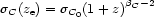(5.9)

and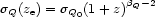(5.10)

where C, and Q apply to RM generated in any intervening clouds, and that associated with the quasar, respectively. The subscript 0 refers to the present epoch, as before.

Welter et al (1984), using these concepts, developed appropriate general mathematical formalisms which can be used to deduce, or limit the parametersC, A,C,Q and. They show that the measured2(ze), which they determined from a sample of 116 quasar RMS indicates significant evolution (namelyC and/orQ > 0), and that, if future RM samples enable a precise specification of the fraction of non-intersections (f0), it should be possible in future to specify all of the above unknowns. This information, together with future high resolution absorption spectra for the same quasars, will provide fairly direct data on the evolution of Faraday rotating intervenors. out to the most distant quasars. This is an exciting prospect, in that it can potentially generate magnetic field estimates over > 90% of the Hubble time.

The existence of a significant fraction (f0) of quasars which do not intersect an intervenor was indicated in the analysis of Kronberg and Simard-Normandin (1976). It is additionally interesting in that, independent of the `discrete intervenor' properties discussed here, improved precision in the measurement of extragalactic RMs may in future either lower the limit of null detection of a widespread IG magnetic field, or possibly detect such a field, if |Bigm|010-8.5 G (section 5.1).